# Review Lesson: Fractions, Word Problems and Patterns.

These 4 problems actually took a whole period! Problem-solving takes time.

CW review

We did this review in groups of 3, with some students rotating after each problem, but the rotation is not necessary. (See Alpha Beta Gamma post from Sept) Rotation breaks up the period with a little movement, and decreases group competition.

### Problem #1: Claire has 4 cookies more than Max. Max has 3 times as many cookies as Oliver. Altogether they have 81 cookies. How many does Claire have?

VERDICT: The kids have got it now.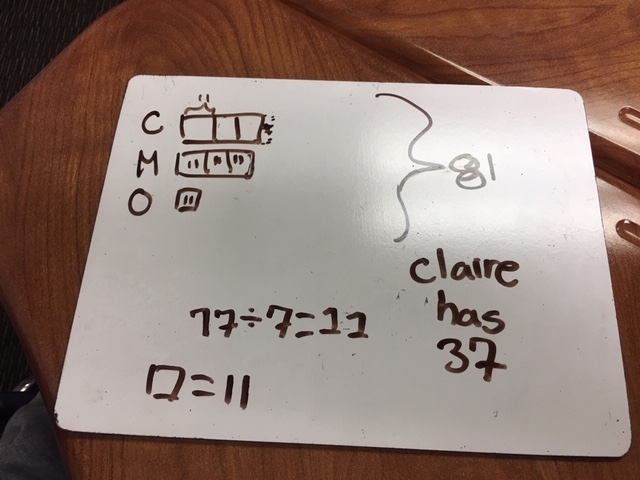###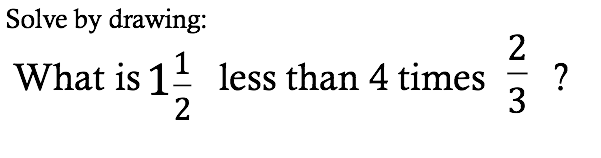VERDICT: The 2-step nature of this problem threw the students off. They could do 4 times 2/3 (by drawing 2/3 four times) and get eight thirds, but most couldn’t figure out how to find 1 and 1/2 less. Several could do 8/3 – 3/2 algorithmically, but could not draw it. We finally collaborated on the visual: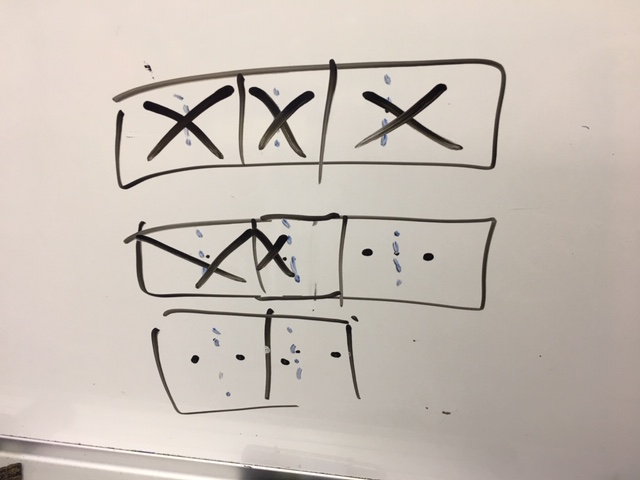Answer by counting up 6ths:

7 sixths.

###VERDICT: Surprisingly, most students got this, even the “nth” challenge. This pattern analysis was not expressly “taught” – it seems to have grown through exposure and observation.### Problem #4:  Ahhh…. puzzling.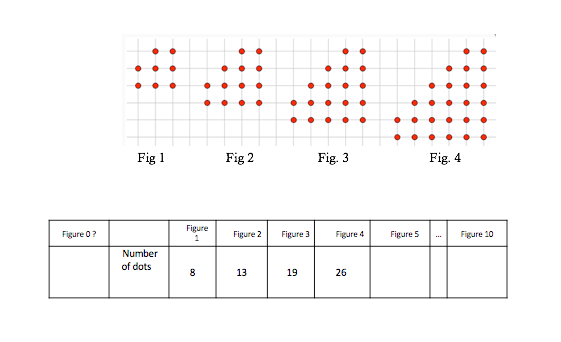VERDICT:  There was a lot of buzz around this problem. Most of us just wrote out the first 10 dot numbers, adding incrementally one more each time.  (Everyone saw the +5, +6, +7, +8 pattern.  So the tenth figure has 89 dots.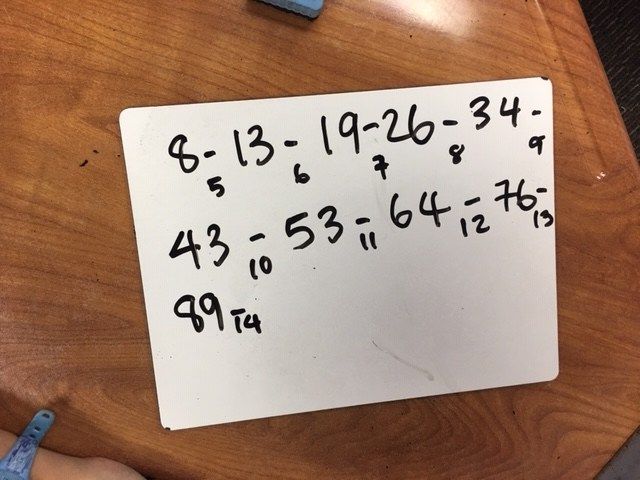But one pair of girls saw this pattern:

Figure 1:   Start at the bottom with 2 rows of  THREE, which is the fig. # + 2. Then add 2.

Figure 2:  Start at the bottom with 2 rows of  FOUR, which is the fig. # + 2. Then add 3 +2

Figure 3:  Start at the bottom with 2 rows of  FIVE, which is the fig. # + 2. Then add 4+3+2

So Fig. 10 is  –>    2 rows of  TWELVE (which is the fig. # + 2). Then add 9 + 8 + 7 + 6 + 5 + 4+ 3 +2

WOW!

## AND THE CHALLENGE?

Two or three students in each class determined to figure out the rule for the nth term.  I was not expecting this, and had to (secretly) use my algebra to determine that it is.

Therefore, we thought it would serve as a problem that gives our fastest students some challenge. They should get a chance to feel confused, just like everyone else! They should learn that some problems are just plain hard, and take perseverance.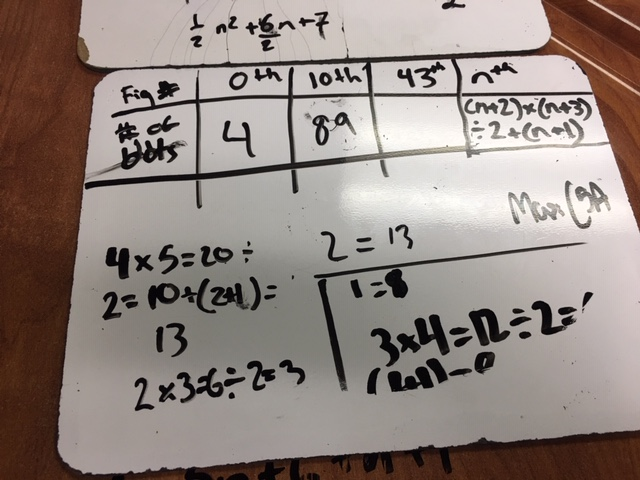Surprise, though. In both classes, students found a solution! Here is one of them: (Top right above):   [(n+2)(n+3)] ÷ 2 + n + 1.  This actually multiplies out to the quadratic function  that we got.

One student tried to explain it to me; here is the video, but I have don’t really understand his logic. (5th graders don’t have a lot of precise math language yet, but that doesn’t slow them down!) Enjoy: